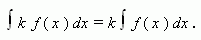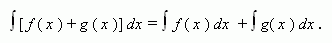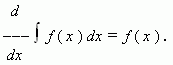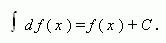# Basic properties of indefinite integral

If  a function f ( x )  has a primitive on a segment X , and k – a number, thenIf  functions f ( x )  and g ( x ) have primitives on a segment X , thenIf  a function f ( x )  has a primitive on a segment X , then for interior points of this segment:If f ( x )  is a continuous function on a segment X and differentiable in interior points of  this segment, then: